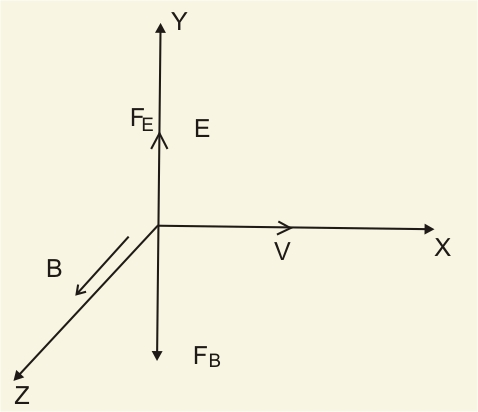# A particle of charge q and mass m is moving with velocity in the positive x-direction.(a) It is subjected to a uniform magnetic field B directed along negative z-direction. Explain briefly the trajectory it would describe.(b) When the particle is subjected simultaneously to both the magnetic and electric fields directed along the z-axis and y-axis respectively, obtain the condition when the particle will go undeflected.

a) Here the velocity of particle  is along the positive x - direction. The magnetic field is along the negative z-direction.

The force experienced by the particle is

So the direction of F is found by applying screw rule or the right-hand thumb rule. It is along the positive y-direction. So the particle makes a circular path, where the radius of the path is

or

b)Here the particle is subjected simultaneously to both magnetic and electric field. So the force experienced is

Here the condition is Electric field and Magnetic field are perpendicular to each other and the velocity of the particle is perpendicular to both.

Then, we have,

(from figure)

So

Therefore,

Thus the electric force and magnetic force are in the opposite direction. So to obtain the condition that the particle will go undeflected adjust the two forces to make magnitude equal. Then total force acting on the particle is zero.

That is

Which means when the particle have velocity , the particle will go undeflected.

### Preparation Products

##### Knockout NEET Sept 2020

An exhaustive E-learning program for the complete preparation of NEET..

₹ 15999/- ₹ 6999/-
##### Rank Booster NEET 2020

This course will help student to be better prepared and study in the right direction for NEET..

₹ 9999/- ₹ 4999/-
##### Knockout JEE Main Sept 2020

An exhaustive E-learning program for the complete preparation of JEE Main..

₹ 12999/- ₹ 6999/-
##### Test Series NEET Sept 2020

Take chapter-wise, subject-wise and Complete syllabus mock tests and get in depth analysis of your test..

₹ 4999/- ₹ 2999/-